[BACK]
 Intelligent Automation & Soft ComputingDOI:10.32604/iasc.2022.023240Article

Modified Optimization for Efficient Cluster-based Routing Protocol in Wireless Sensor Network

1College of Computing and Informatics, Saudi Electronic University, Riyadh, 93499, KSA
2Self-Development Skills Department, Common First Year Deanship, King Saud University, Riyadh, 11451, KSA
*Corresponding Author: Marwah Mohammad Almasri. Email: m.almasri@seu.edu.sa
Received: 31 August 2021; Accepted: 01 December 2021

Abstract: Wireless Sensor Networks (WSN) comprise numerous sensor nodes for monitoring specific areas. Great deals of efforts have been achieved to obtain effective routing approaches using clustering methods. Clustering is considered an effective way to provide a better route for transmitting the data, but cluster head selection and route generation is considered as a complicated task. To manage such complex issues and to enhance network lifetime and energy consumption, an energy-effective cluster-based routing approach is proposed. As the major intention of this paper is to select an optimal cluster head, this paper proposes a modified golden eagle optimization (M-GEO) algorithm to figure out the most significant issue of choosing an optimal cluster head in every cluster. The M-GEO algorithm selects an optimal cluster head among all the sensors by employing diverse factors namely the residual energy, node degree, distance among the nearby sensors, centrality of sensor nodes as well as distance between the cluster head and sink node. Additionally, the yellow saddle goatfish (YSG) optimization algorithm is employed in generatingan optimal routing path from the cluster head to the base station. Also, the YSG optimization algorithm detectsthe shortest routing path thereby minimizing the energy consumption. Then later, the performance analyses for various parameters are performed to evaluate the performance of the proposed approach.

1  Introduction

Wireless sensor networks (WSNs) are largely been reviewed in a ubiquitous computational environment due to their extensive usage . The WSN finds applications in diverse domains like congestion control, biomedical applications, health care monitoring, home automation, environmental management, military and surveillance applications. Generally, WSN contains several thousand and hundreds of sensor nodes that are widely distributed for sensing, evaluating and receiving the information as well. The WSN sensor nodes are of reasonable cost, easily affordable and contain a high capacity to sense, process and transmit the data . In addition to this, the WSN sensor nodes are facilitated with memory, processor, data storage device and radio-transmission module. On the other hand, a huge resource constraint is necessary to design the above-mentioned components. One of the most significant constraints of WSN is its limited energy source. Hence, energy-saving is considered a complicated issue that requires investigation for enhancing the network lifetime . Numerous issues have been investigated to enhance WSN that includes clock synchronization approaches, efficient routing protocols, secure data aggregation approaches, medium access control approaches as well as lo power and energy consumption devices .

Numerous sensor network models permit the sensors for transmitting the data to the base station or sink using a multi-hop routing process. In general, the WSN routing protocol determines the path among the source node and the base station (i.e., destination node) to transmit the sensed data. The WSN efficiency relies mainly on routing that promptly influences the network lifetime . The significant role of routing protocol involves the enhancement of both network lifetime and reliability by considering the sensor node capability containing various resource constraints namely low communicated bandwidth, limited power as well as slow processor . Minimizing communication overhead to transmit the data and to determine the optimal routing path is considered a challenging issue and it must be taken into consideration. The most well-known and commonly utilized approach for routing protocol is the clustering approach .

The cluster-based routing protocol provides an effective way of minimizing the energy consumption and the total number of messages transmitted to the base station or the sink node. The clustering approach also effectively manages the scalability and energy consumption of the network to enhance the network lifetime . The clustering approach of the WSN partitions the networks into diverse sensor groups referred to as clusters. The cluster head (CH) is selected as a leader by the sensors from every cluster using centralized control algorithm. The sensors gather data from every cluster and transmit it to the respective cluster head. The two different types of cluster head communication are inter-cluster communication and intra-cluster communication . The transmitted data are later received by the cluster head and transmitted to the sink node. The clustering sensor provides numerous benefits for the effective management of WSN. It also permits power saving in sensors and therefore the CH eliminates the false positive and redundant data using the aggregation method . The sensor node turns to be faulty and unreliable because of nodal deployment in the challenging environment. During packet transmission, the average energy consumption is considered a challenging problem and due to insufficient energy, there occurs packet drop in data transmission. This paper considers both distance and energy to develop energy-efficient WSN since the energy consumption of every node depends significantly on distance among the nodes. In order to minimize the packet losses, every nodal energy in WSN must be taken into consideration. The objective function discussed in this routing process is residual energy, distance among the sensor nodes, distance between base station and cluster head, node centrality and node degree. This research paper fulfills the following objectives.

•   Proposing modified golden eagle optimization (M-GEO) algorithm for optimal selection of cluster head from every cluster.

•   Utilizing yellow saddle goatfish (YSG) optimization algorithm to generate the optimal routing path from the cluster head to the base station thereby detecting the shortest routing path and minimizing the energy consumption.

•   Enhancing the network lifetime and reducing the energy consumption of the nodes during data packet transmission.

The remaining of the paper is structured as follows: In Section 2, the latest surveys based on clustering approaches are presented. Section 3 section portrays the ongoing challenges of WSN and objective function. The system model containing network design, energy model and distance model is described in Section 4. Section 5 depicts the proposed approach for optimal CH selection and route generation. The experimental evaluations are discussed in Section 6. Section 7 concludes the article.

2  Theoretical Backgrounds on Cluster based Routing Protocols

Numerous research papers have been implemented to obtain energy-efficient cluster-based routing protocols for wireless sensor networks. A detailed review of few relevant research works is discussed in Tab. 1.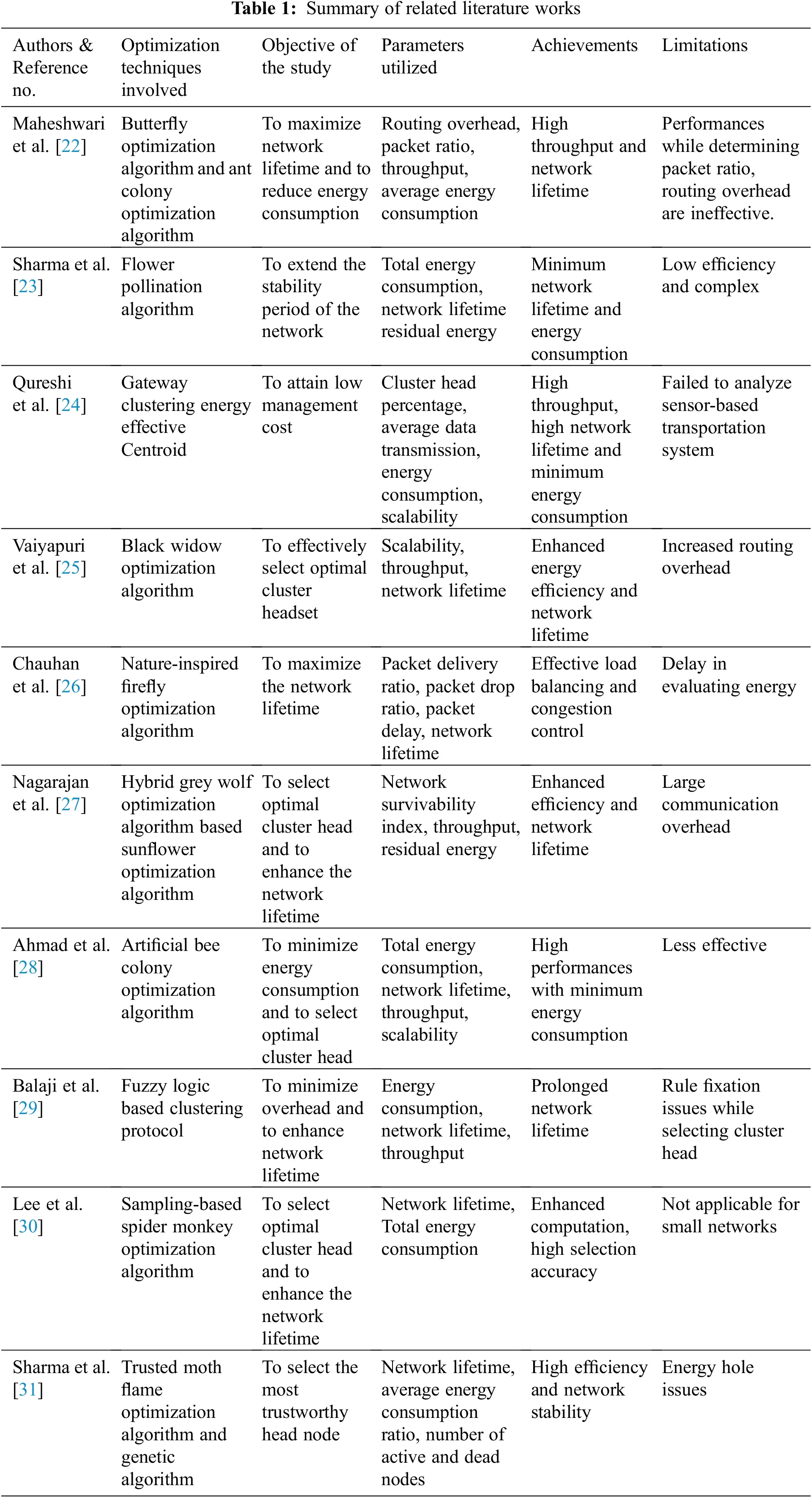Maheshwari et al.  established a concept based on an energy-efficient cluster-based routing protocol for WSN. This approach utilized two different types of optimization algorithms like butterfly optimization algorithm and the ant colony optimization algorithm to maximize the network lifetime. Various simulation measures namely routing overhead, packet ratio, throughput, and average energy consumption were utilized to obtain minimum energy consumption with high throughput rate. But ineffective system performances were regarded as the major drawback.

An energy-efficient stable clustering approach for WSNsemploying flower pollination optimization algorithm is proposed by Sharma et al. . The major intention of this approach was to extend the stability period of the network. The experimental investigation was conducted and from the analysis, it was clear that energy consumption was minimized and the network lifetime was enhanced. But low efficiency and complexity were considered as the disadvantage of this approach.

Qureshi et al.  demonstrated an optimized cluster-based dynamic energy-aware routing protocol for wireless sensor network in the field of agriculture. This approach utilized gateway clustering energy-effective Centroid to achieve low management costs. Cluster head percentage, average data transmission, energy consumption and scalability are the parameters employed for simulation. The throughput rate and network lifetime were enhanced but this approach failed to analyze sensor-based transportation systems.

A Novel Hybrid black widow optimization algorithm for Cluster-Based Routing Protocol in IoT Based Mobile Edge Computing was proposed by Vaiyapuri et al. . This approach was mainly developed to effectively select an optimal cluster headset. The results of this approach obtained enhanced energy efficiency and network lifetime. High routing overhead was the significant drawback of this approach.

Chauhan et al.  utilized nature inspired firefly optimization algorithm for the mobile sink-based energy efficient cluster head selection strategy for wireless sensor networks. Few parameters namely packet delay, packet drop ratio, packet delivery ratio, and network lifetime were employed to obtain effective congestion control and load balancing. On the other hand, there occurred a delay during energy evaluation was the major shortcoming of this approach.

An energy-efficient cluster head selection in wireless sensor networks for lifetime enhancement using hybrid grey wolf optimization algorithm based sunflower optimization algorithm was demonstrated by Nagarajan et al. . Network survivability index, throughput and residual energy were the parameters utilized to enhance the efficiency and network lifetime. Due to large communication overhead, this approach was ineffective.

An energy-efficient cluster head selection using artificial bee colony optimization for wireless sensor networks was developed by Ahmad et al. . This approach was mainly intended to minimize overhead and to maximize the network lifetime. Total energy consumption, network lifetime, throughput, scalability were the parameters utilized to achieve high performances with minimum energy consumption. The overall effectiveness of this approach was low when compared with other approaches.

Balaji et al.  utilized a fuzzy logic controller to develop an energy efficient cluster routing protocol to maximize the lifetime of wireless sensor networks. The major intention of this approach was to minimize overhead and to enhance network lifetime. Throughput, Energy consumption, and network lifetime were the performance measures employed to determine the performance of the system. The network lifetime of this approach was prolonged but there occurred rule fixation issues while selecting cluster head.

Energy-Efficient cluster-head selection for wireless sensor networks utilizing sampling-based spider monkey optimization was proposed by Lee et al. . Here, a sample based spider monkey optimization algorithm was employed in selecting optimal cluster head and to enhance the network lifetime. The experimentation was conducted and the results revealed that the selection accuracy was very high; however this approach was not applicable for small networks.

Sharma et al.  developed a trusted moth flame optimization algorithm and genetic algorithm to provide a secure and energy-efficient cluster head selection. Network lifetime, average energy consumption ratio, number of active and dead nodes were the parameters utilized to select trustworthy mode with high efficiency and network stability. On the other hand, energy hole issues were regarded as the significant drawback of this approach.

3  Problem Descriptions and Objective Function

This section portrays the ongoing challenges of WSN and in what way the proposed approach addresses these issues. Also, the objective function to provide an energy-efficient approach is discussed.

3.1 Problem Formulation

A suitable selection of fitness functions must be taken into consideration to create optimal energy efficiency in WSN. Two different energy approaches namely weighted energy-efficient clustering-based routing protocol and aware cluster-based routing protocol approach provide great importance to the residual energy of the sensor node. The network energy consumption minimizes only if these approaches provide the same level of priority regarding distance and energy. In few other routing and clustering techniques, the WSN performances were influenced when numerous non-cluster head members are present in the cluster head. Furthermore, the performances are also affected due to nodal density. It is also worth noting that in WSN, the direct transmission of data among the cluster head as well as sink or base station consumes more amount of energy that further results in packet loss among the networks. The sensor node turns to be faulty and unreliable because of nodal deployment in the challenging environment. During packet transmission, the average energy consumption is considered a challenging problem and due to insufficient energy, there occurs packet drop in data transmission.

This paper considers both distance and energy to develop energy-efficient WSN since the energy consumption of nodes depends significantly on the distance among the nodes. Thus, the energy consumption is in direct proportion with the distance among the sensor nodes. Furthermore, every nodal energy in WSN is taken into consideration to minimize packet loss. Here, the M-GEO algorithm is employed in selecting the optimal cluster head and the YSG algorithm is utilized to develop an optimal route to the destination node from the source node. The objective functions discussed in this routing process are residual energy, distance among the sensor nodes, distance between the base station and cluster head, node centrality and node degree. Thus, an energy-efficient approach is applicable for both small and large-scale applications by considering the above-mentioned parameters. This further minimizes the packet loss among the networks.

3.2 Objective Function Formulation

This section depicts the mathematical formulation of five objective functions namely residual energy, distance among the sensor nodes, distance between base station and cluster head, node degree and node centrality.

Objective 1: CH Residual Energy

In WSN, several tasks are performed by the CH (i.e., data collection from ordinary sensor nodes and transmitting data to the sink). To accomplish the above-mentioned tasks, high energy is required by the CH. Therefore, a CH prefers a sensor node containing high residual energy.

F(1)=MaximumJ=1N1RJ (1)

where RJ signifies the residual energy of Jth CH .

Objective 2: Node Degree

Node degree refers to the total number of node that belongs to the corresponding CH. The CH containing the minimum number of sensor nodes is selected. This is because more energy is lost when the total number of CH is increased.

F(2)=MinimumJ=1NSJ (2)

From Eq. (2), the sensor nodes belong to the CH is SJ .

Objective 3: Distance between the Sensor Nodes

The distance among the nodes refers to the distances among the CH and ordinary sensor nodes. The dissipation of nodal energy depends mainly on the transmission path distance. When the transmission distance towards the sink is less, then the energy consumption of the sensor nodes is small. Thus,

F(3)=MinimumJ=1N[J=1SJD(δJ,CHK)SK] (3)

where D(δJ, CHJ) indicates the distance between the Jth sensor and Kth CH.

Objective 4: Centrality of Sensor Nodes

Node centrality refers to, what extend a node is located centrally from the neighboring sensor nodes. Therefore, the mathematical formula to determine the centrality of the sensor nodes is obtained as follows.

F(4)=MinimumJ=1N[KMD2(J,K)/M(J)N] (4)

From Eq. (4), M(J) and N denotes the total number of neighbor sensor nodes and network dimension respectively .

Objective 5: Distance among the Sink and CH

It defines the total distance between the CH and sink. The energy consumption of the sensor node relies mainly on distance utilizing a transmitting path. Let us consider an illustration; if both the CH and sink are far away from each other, more energy is required for transmitting data. This results in an abrupt drop and large energy consumption of CH. Therefore, the sensor nodes containing less distance from the sink are chosen while transmitting the data . Hence,

F(5)=MinimumJ=1ND(CHK,sink) (5)

where the total distance between the CH and sink is D(CHK, sink).

4  System Design

This section illustrates the comprehensive description regarding three different designs namely the network design, energy design and distance design.

4.1 Network Design

See Fig. 1 depicts the WSN structure comprising sensor nodes, CH, base station, transmission link among CH and sensor nodes and CH as well as the base station. In general, the WSN relies on five different features namely the topological features, sensor allocation, energy consumption, data sensing as well as radio communication . The sensors are located either manually or randomly based on certain applications like military, industrial and environmental domains for monitoring and controlling. Fig. 1 comprises a multi-hop network which can be obtained in a graphical form G stated below.

GP={δ,ψ} (6)

where the base station and the sensor nodes are represented by δ. ψ indicates the transmission link between various sensor nodes. The following assumptions made for the network are mentioned below.

(i)   A 2D Cartesian system is employed for the deployment of random sensors

(ii)   Well-equipped non-rechargeable power resources are utilized for all sensor nodes

(iii)   The sensor nodes are not feasible to vary their location after they are deployed

(iv)   The processing and communication abilities of all sensor nodes are analogous

(v)   The sensor nodes comprising initial energy are identical

(vi)   The transmission link between sensor nodes is bi-directional (i.e., capable of diffusing data in both directions).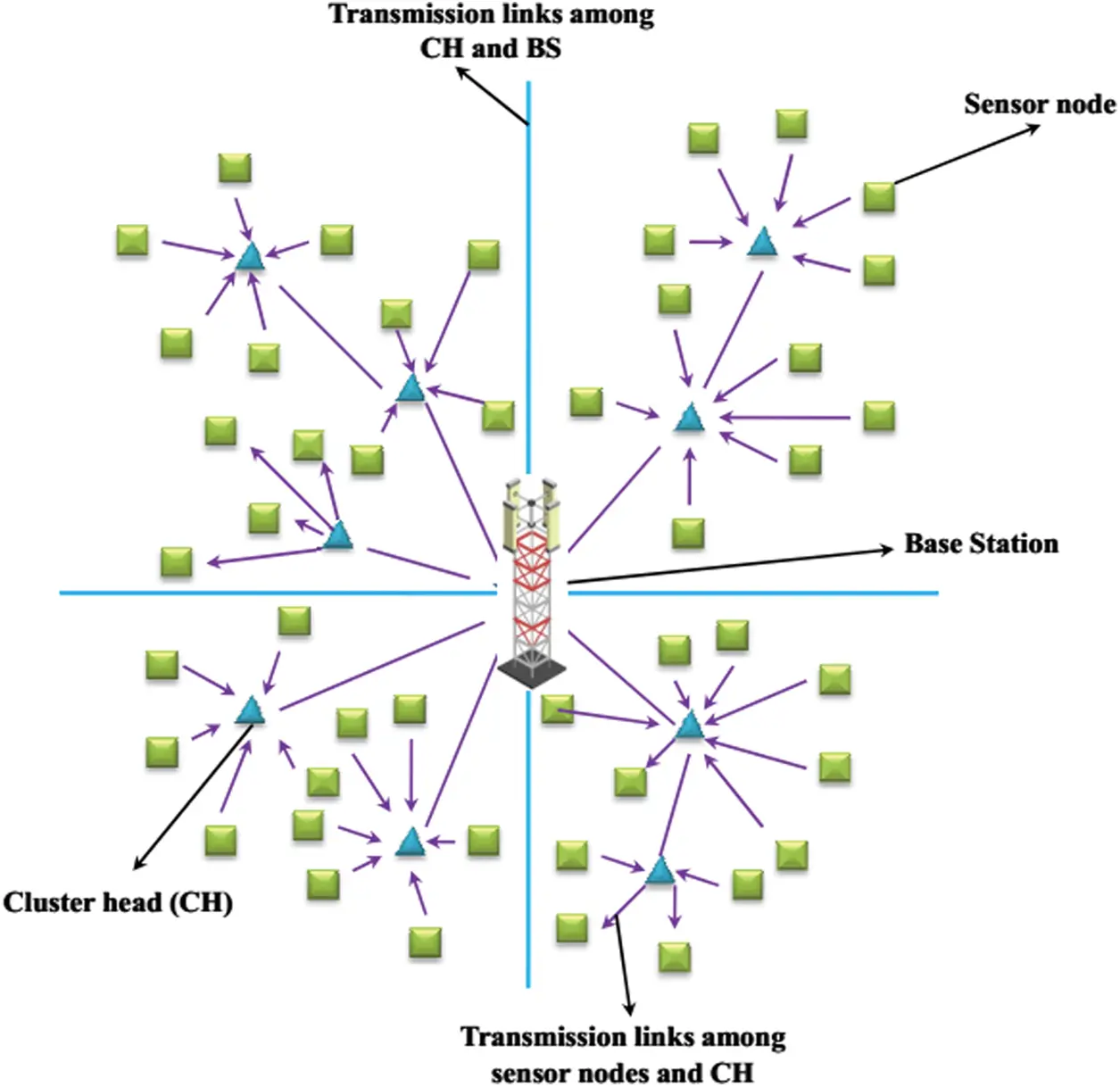Figure 1: System model of WSN

4.2 Distance Design

In general, the selected CH of a particular network transmits messages to a state wherein they operate as CH . Under such circumstances, every sensor node evaluates the specific distances from CH. The node in the network is clustered again with selected CH employing a distance matrix D (m*n) stated in Eq. (7).

D(mn)=[EX(1)EX(2)EX(N)EX(1)EX(2)E,X(N)EX(1)EX(2)EX(N)] (7)

From Eq. (7), E indicates the Euclidean distance between the CH. X(1) toX(N) is the sensor nodes. Let us consider two sensor nodes Yth and Zth containing the p and q respectively. Eq. (8) provides the mathematical formula to determine the Euclidean distance . Thus,

E(Y,Z)=(YPYQ)2+(ZPZQ)2 (8)

4.3 Energy Design

In this paper, the receiver and the transmitter energy are computed using a basic first-order ratio design . The energy consumed in collecting and transmitting k bit packets along the distance D is obtained in Eqs. (9) and (10).

ϵT(k,D)={k×ϵE+k×ϵF×D2ifDD0k×ϵE+k×ϵM×D4ifD>D0 (9)

ϵR(k,D)=k×ϵE (10)

The receiving and the transmitting energy dissipated is denoted by εE. D0 indicates the threshold distance. The following equation determining the threshold value is stated in Eq. (11).

D0=ϵFϵM (11)

From the above Eq. (11), εF and εM denotes the amplification energy of free space and multipath. It is also noted that the transmitter amplification model is dependent on both εF and εM .

5  Proposed Methodology

Fig. 2 depicts the proposed workflow. The proposed approach comprises two major phases: (a) optimal CH selection (b) Routing process. The modified Golden eagle optimization algorithm is employed in selecting the optimal cluster head. M-GEO algorithm selects an optimal routing path among the CH and sink node or base station. Then later the CH transmits the data to the sink node via the path generated using the YSG algorithm.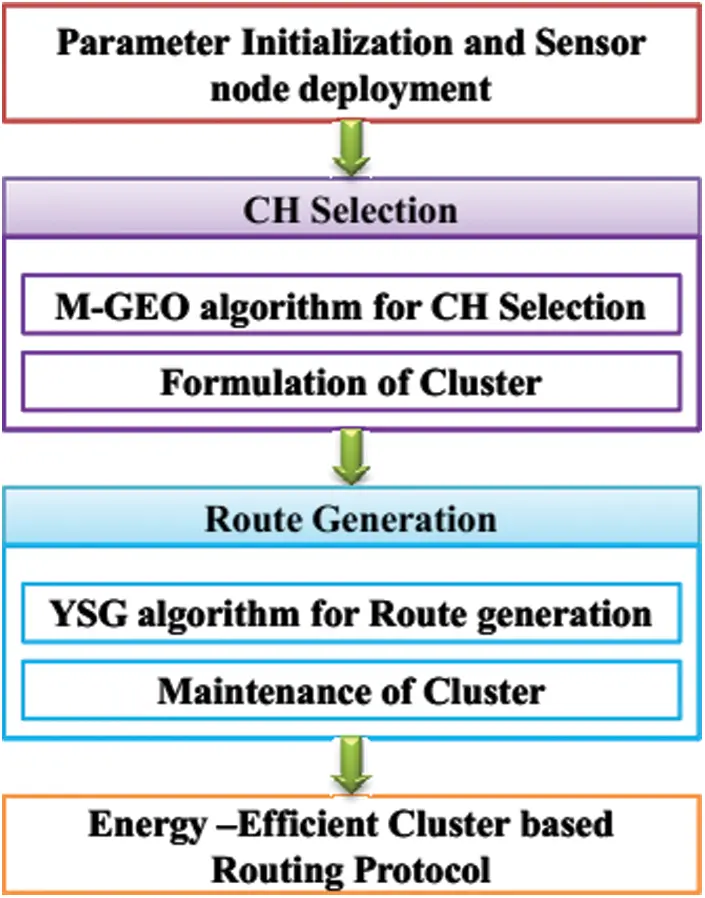Figure 2: Proposed workflow

5.1 Modified Golden Eagle Optimization (M-GEO) Algorithm for CH Selection

This paper proposes a modified golden eagle optimization (M-GEO) algorithm to figure out the most significant issue of choosing an optimal CH in every cluster. In WSN, every sensor node is considered as an individual golden eagle and their prey selection process depends upon the distance among an individual sensor node and the nearby sensors. Here, the sensor nodes are attracted by the prey selection process of the golden eagle. The performance behavior and the characteristics of the golden eagle are based on search algorithmic processes. The M-GEO algorithm selects an optimal CH among all the sensors by employing node degree, residual energy, the distance among the nearby sensors, centrality of sensor nodes as well as distance among the CH and sink node. The steps involved in the M-GEO algorithm for optimal CH selection are discussed in the following section.

5.1.1 M-GEO Algorithm

The generic name of the golden eagle is Aquila and the specific name is chrysaetos belongs to the family of Accipitridae and species of hawks and eagles. Generally, the golden eagles are specialized hunters with extraordinary vision capability and influential talons. The golden eagles can fly at a speed of 190 km per hour and are distributed widely in the northern hemisphere of the earth. Hunting and cruising are the two unique characters of golden eagles that are performed in a spiral path. Additionally, the golden eagles cleverly establish a balance for snatching the best kill at a reasonable amount of energy and time. One of the special features of this eagles are it lower the altitude gradually and at the same time, it approaches the prey. The mathematical modeling and the step-by-step procedure involved in the M-GEO algorithm are discussed as follows .

Circular Movement of Golden Eagle

The M-GEO is based on the circular movement of golden eagles. Each golden eagle remembers the best spot it has traveled previously. Simultaneously, the eagle attacks the prey and cruise for searching for better foodstuff. For every iteration, every golden eagle J chooses the prey randomly of a new golden eagle G. Then later it makes a circular movement for identifying the best spot visited before by a golden eagle G. Additionally, the golden eagle J also can select to circle and recollect the memories. Hence,

G{1,2,P} (12)

Eq. (12), P signifies the population size .

Selection of Prey

The golden eagle optimization algorithm utilizes the prey selection process of the chameleon optimization algorithm . Similar to other creatures, the chameleons wander around the trees and in the desert in search of prey. Chameleons are assimilating to climb and hunt with clear eyesight where they can view the prey upto 32 feet. The chameleons significantly nourish themselves by drizzling their clingy tongue to catch their prey. The eyes of the chameleons have the proficiency for exploring the search space in locating the prey. The performance activities of chameleons motion during the foraging process is expressed mathematically by updating the positioning strategy stated as follows .

ZTJ,K={ZtJ,K+A1(PtJ,KQtK)+A2(QtKPtJ,K)JCZtJ,K+α[(UKLK)3+LK]S(0.5)J<C (13)

From Eq. (13), the current and new position of the Jth chameleon is represented by t and T. A1 and A2 is the two different positive numbers for controlling the exploration capability. The best and the global best position scored by the chameleon with respect to Jth dimension and Tth iteration is denoted by PtJ,K and QtK respectively. The random number ranging from 0 to 1 are denoted by J . UK andLK signifies the upper and lower boundaries and the probability of the chameleon perceiving the prey is denoted by C; where C = 0.1. The exploration and exploitation direction is represented by S(0.5) . A parameter α provides the function of iterations.

Exploitation Phase (Attack Process)

The attack phase is designed in such a way that the present golden eagle's position begins with the vector and terminates with the best spot decided by the eagle after memorizing. Therefore, the following equation provides the computation equation of the attack vector .

AJ=ZGZJ (14)

From Eq. (14), the attack vector of the Jth golden eagle is AJ . The best spot visited by eagle G is ZG . The present eagle position with respect to Jth dimension is denoted by ZJ . Because the attack vector directs the golden eagle's population towards the best spot, the exploitation phases of golden eagles are highlighted.

Exploration Phase (Cruise Phase)

In accordance with the attack vector, the cruise vector is computed. The cruise vector is perpendicular to the attack vector and considered as a tangent vector. Therefore, initially, it is necessary to determine the hyperplane H that is computed below .

Z1h1+Z2h2++Zmhm=D,where,H=[h1hm]andz=[Z1Zm] (15)

D=K=1mZKhK (16)

Then the cruise vector with respect to the Jth dimension is determined followed by computing the hyperplane vector. Thus,

K=1maKZK=K=1maKZK (17)

From Eq. (17), the attack vector, design variables and the best spot of the chosen prey are AJ=[a1am],z=[Z1Zm]andz=[Z1Zm] respectively .

Shifting Towards New Spot

The golden eagle displacement generally comprises of two different factors namely the vector and attack. Therefore accordingly the step vector for a golden angle with respect to t iteration is computed in Eq. (18).

ΔZJ=1PaAJAJ+2PcCJCJ (18)

From the above Eq. (18), the random number ranging from 0 to 1 are denoted by 1and2 . The Euclidean norm for both attack and the cruise vectors are AJ and CJ respectively. From Eq. (18),

AJ=K=1maK2 and CJ=K=1mcK2 (19)

Then the eagle position with respect to T + 1th iteration is computed by,

ZT+1=ZT+ΔZJT (20)

The best spot in the memory is worse than the fitness of the new position, and then the memory of the golden eagle is updated with the new position.

Switching Process from Exploration to Exploitation

It is well known that the golden eagles reveal their higher tendency for cruising and hunting at both initial and final stages. In addition to this, the M-GEO algorithm utilizes both Pa and Pc for exploitation and exploration. Thus,

Pa=Pa0+Tt|PatPa0| (21)

PC=Pc0+Tt|PctPc0| (22)

From Eqs. (21) and (22), Pa0,Pat and Pc0,Pct were the first and last values for the tendency to attack and cruise respectively. T and t denote the present iteration and the maximum number of iterations. Thus the above-mentioned steps are the complete process to select the cluster head using the M-GEO algorithm. The following Pseudocode 1 provides the M-GEO implementation for CH selection.5.1.2 Formulation of Cluster

After CH selection using M-GEO, the CH allocates the sensor nodes by employing the potential function stated in Eq. (23).

Sp=B×Residual energy of CHDistance among the CH and sensor (23)

From Eq. (23), Sp and B signifies the sensor node potential and the proportionality constant. Here, the CH allocates the sensors with high residual energy and minimum transmission distance and hence the total energy consumption will be minimal during the transmission of data.

5.2 Yellow Saddle Goatfish (YSG) Algorithm for Route Generation

YSG algorithm is a metaheuristic algorithm stimulated by the performance behavior of the goatfish. In general, the goatfish discovers a hunting strategy to obtain its prey (i.e., small fishes). The YSG algorithm is applied to solve diverse discrete problems that are illustrated in the graphical form containing the number of links and nodes. During the initialization process, each node comprises goatfishes and every individual is accompanied to the weight. Initially, the weights of the links are computed concerning the random value or by employing mathematical expressions.

5.2.1 YSG Algorithm

The collective hunting model of a goatfish is regarded as a guiding principle for developing a bio-inspired algorithm. The YSG algorithm concerns two distinctive types of search agents namely chaser as well as a blocker. One fish in every sub-population is considered as a chaser and the rest are the blockers. The hunting designs of YSG with their necessary steps are discussed as follows .

Initialization Process

Every individual goat fish among the population are generated randomly and distributed uniformly containing lower and upper boundaries of M-dimensional search space area.

PJK=[UKLK]+LK;J=1..NandK=1M (24)

From Eq. (24), the random number ranges from 0 to 1 are represented by . The upper and lower boundaries are UKandLK . PJK signifies the goatfish population .

Chasing Process

During the process of hunting, the prey tries to hide behind the cervices and escape in between the corals. The tactics of the chaser fish involve inserting the barbels into the cervices to catch the prey. Here, the levy flight algorithm is employed in random walk generation. The chaser fish attempts in finding the cervices by varying its position containing random walk. Therefore, the new position of the chaser fish is stated in Eq. (25).

δlT=δlt+βL(α)0<α2 (25)

From the above Eq. (25), the δlT and δlt denotes the current position and new chaser fish position. β,,L()and α signifies the probability distribution function, entry-wise multiplication factor, levy distribution factor and levy index respectively . From Eq. (25),

α=0.001TTMAX/10+1.99 (26)

From Eq. (26), the current generation and maximum iteration numbers are T and TMAX respectively. Every group generally ignores the other sub-populations to obtain the best prey, then.

RS=βL(α)β[UV1/α][δlTδBestT] (27)

From Eq. (27), the best chasing fish obtained so far is denoted by δBestT and RS signifies the random step. U and V denotes the normal distribution factor. Then the new position value with respect to Eq. (25) can be written as,

δlT+1=δlT+RS (28)

Blocking Process

Once the chase fishes are selected for every cluster, the rest goat fishes are considered as the blocker. During the blocking process, the hunter strategy is employed to encircle the corals thereby block the escaping prey. The blocker fish moves in a circular motion when the chaser fish approaches the prey. Therefore, the new position of the blocker fish is evaluated based on the logarithmic spiral path stated below .

δgT+1=dg.EBλ.Cos2πλ+δl (29)

From Eq. (29), λ denotes the random value ranging from (r, 1). B signifies the constant determining the direction and shape of the spiral path. The distance among the current position of both chaser and blocker fish is denoted by dg.

Swapping of Roles

The significant intention of the blocker fish prevents the perspective of the prey. The prey progresses to the hunting location during hunting. Hence, the blocker fish which is near to the prey show the way to hunt thus become the new chaser fish and the present chaser fish becomes the blocker fish. Such a technique is referred to as the swapping of roles.

Zone Transformation

The group changes its position for identifying the new prey once the area has been exploited completely. Under such circumstances, the YSG algorithm utilizes an exploitation parameter and hence for each cluster, if pre-determined iterations exceed without determining the optimal solution, then it is regarded as successful hunting. Therefore zone transformation is performed for entire goat fishes.

PgT=δBest+Pgt2 (30)

From Eq. (30), the new position of the goatfish is PgT . PgtandδBest denotes the best position of the goatfish and the best fish among the cluster. The current position of the goatfish is denoted by Pgt . The above-mentioned procedure provides the routing process among the base station and source nodes are discussed in the following Pseudocode 2.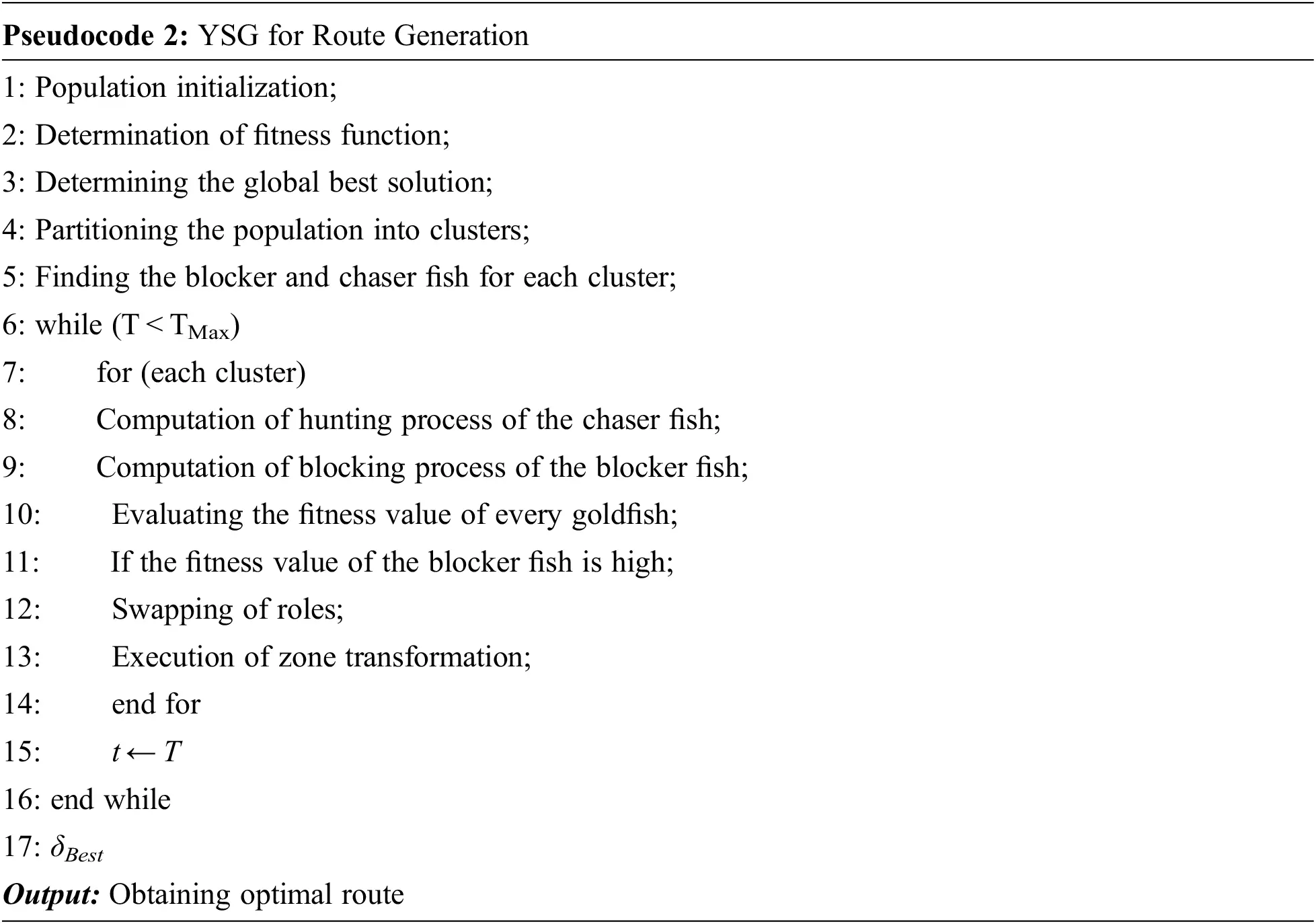5.2.2 Maintenance of Cluster

In this paper, the maintenance of clusters is the most significant phase for balancing the load among the cluster. Due to inter-cluster congestion, the neighboring cluster present near the sink node or the base station consumes more energy. Hence, maintaining clusters is necessary for eliminating the failure of nodes. This results in the enhancement of network lifetime during the transmission of data from the source node to the base station. Furthermore, if the residual energies of the CH exceed the threshold level, then M-GEO algorithm is initialized for network clustering. Later, the clustering M-GEO algorithm selects the CH and YSG algorithm is employed in the route generation process.

In the proposed approach, the CH is selected effectively using the M-GEO algorithm. The CH selection is based on employing node degree, the distance among the nearby sensors, residual energy, centrality of sensor nodes as well as distance between the CH and sink node. The base station or the sink node frequently monitors the residual energy to prevent nodal failure while transmitting the data. Consequently, the YSG algorithm is employed to generate an optimal routing path from the CH to the base station. It is capable of detecting the shortest routing path to minimize energy consumption. Therefore, the M-GEO and YSG algorithm for optimal selection of CH and route generation is designed to obtain energy-efficient WSN. Thus, energy-effective WSN is employed in enhancing the total number of packets and network lifetime while transmitting the data.

6  Experimental Evaluation

This section evaluates the performance analysis of the proposed approach. The major intention of this paper is to maximize the network life and to reduce the overall energy consumption of the network. The evaluation results and comparative analysis to achieve an energy-efficient proposed approach are discussed in the following section.

6.1 Experimental Configuration

The proposed energy-efficient routing protocol approach is investigated and executed under the platform of MATLAB R2018a, windows 8 OS containing 4 GB RAM and Intel core i3 processor. The significant intention of utilizing MATLAB is to obtain simple mathematical formulations and appropriate analysis of data.

6.2 Parameter Description

Tab. 2 depicts the experimentation parameters employed in this experiment containing the deployment area, nodes, clusters, initial nodal energy, packet size, etc. Tab. 3 describes the parameters of the M-GEO algorithm and YSG algorithm.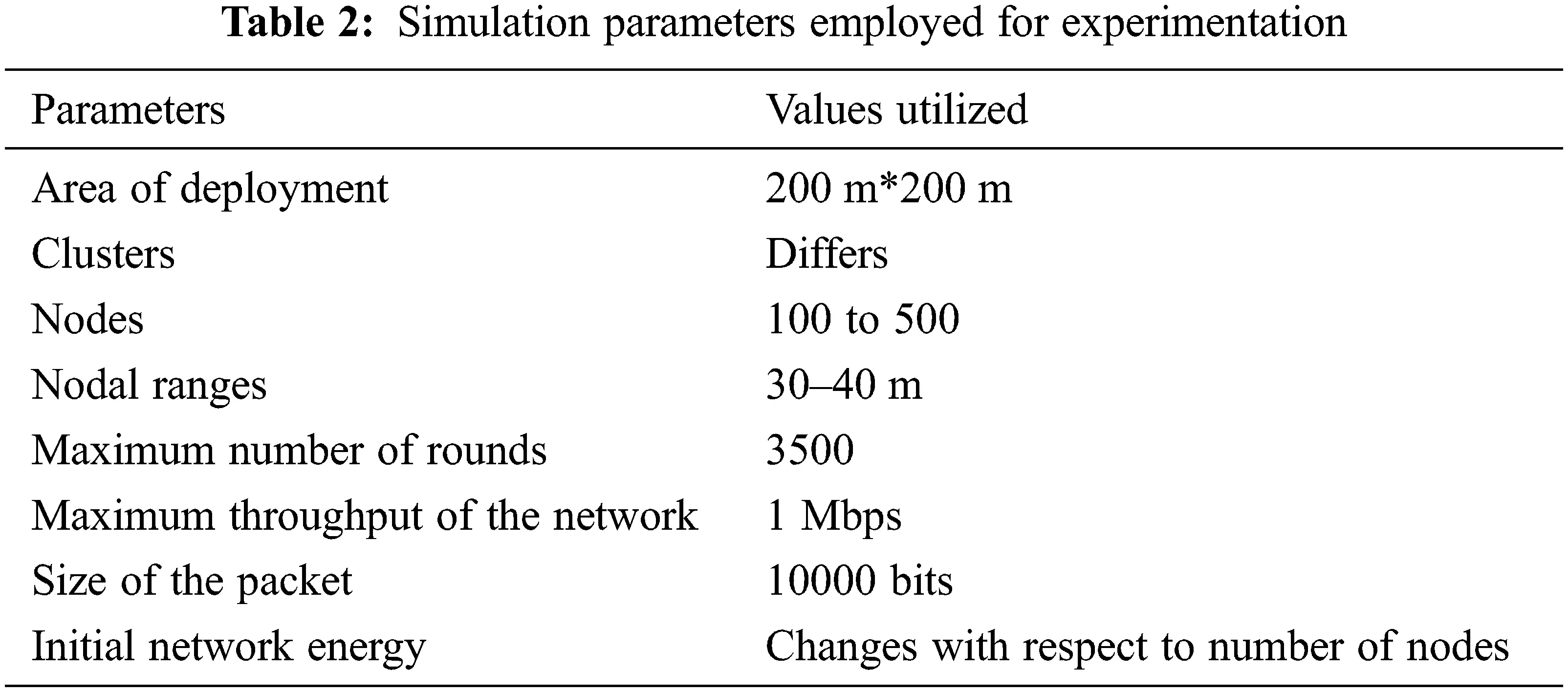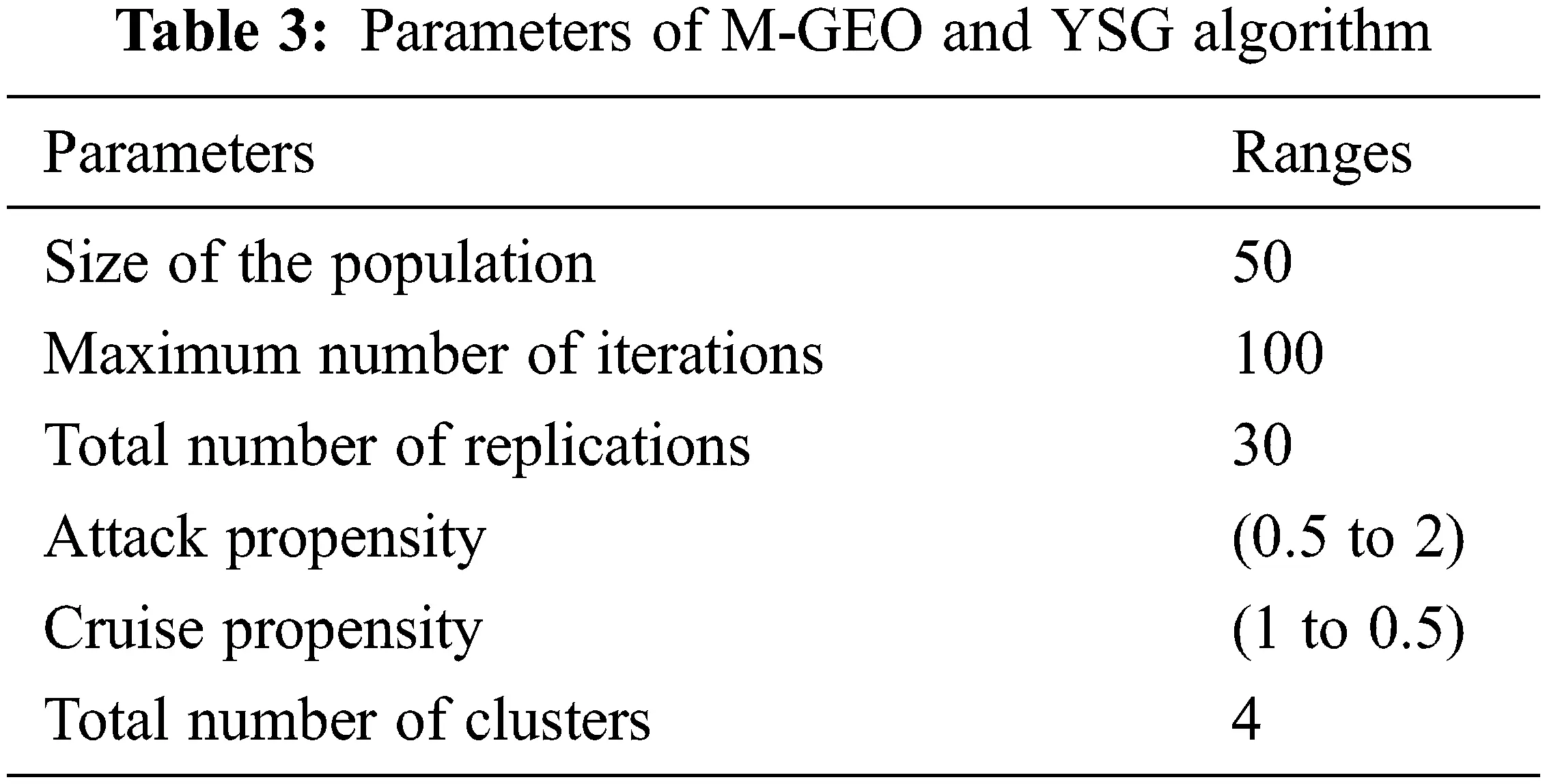6.3 Performance Measures

In this section, various parameters namely the network lifetime, number of alive nodes, energy consumption, throughput, number of dead nodes, packet delivery ratio as well as routing overhead is described.

The network lifetime signifies the total rounds and total time duration of the network for performing the operations. In other words, it defines the rounds that the nodes will die during the task processing. Eq. (31) provides the mathematical formulation of the network lifetime.

From the above Eq. (31), CM, LJ(SN) and NK signifies the coverage matrix, source node lifetime as well as the total number of nodes. From Eq. (31),

CM(JK)={1if source node monitors the target0otherwise (32)

6.3.2 Number of Alive Nodes

It refers to the sensor node in the network containing adequate energy to process its task. The mathematical expression involved in determining the total number of alive nodes is stated in Eq. (33).

AN(J)=RE(N)0 (33)

From the above Eq. (33), the total number of alive nodes and the energy of the node with respect to the Jth round are AN(J) and RE(N) respectively.

It refers to the sensor node in the network that doesn't contain adequate energy to process its task. The mathematical expression involved in determining the total number of alive nodes is formulated in the below Eq. (34).

DN(J)=RE(N)0 (34)

From Eq. (34), the total number of dead nodes is DN(J).

6.3.4 Energy Consumption

The mathematical formulation to determine the total amount of consumed energy by the sensor nodes is formulated in Eq. (35).

EC=E(CH)+E(CM) (35)

From the above Eq. (35), EC,  E(CH) and E(CM) signifies the energy consumption, energy employed by the cluster head and cluster member.

6.3.5 Throughput

Throughput is the ratio of the product of total number of packets and the size of the packet to the total time taken during transmission of data. The mathematical formula to depict the throughput value is determined in Eq. (36).

TR=PsentPsizeT (36)

From the above Eq. (36), PsentandPsize denotes the total number of packets sent and packet size respectively. The throughput value and the time was taken are denoted by TR and T.

6.3.6 Packet Delivery Ratio

The term packet delivery ratio refers to the fraction of the total number of received packets by the total sent packet. Thus,

The term routing overhead refers to the ratio among the total packet received by the sink node to the total number of packets generated.

6.4 Performance Analysis

Fig. 3 presents the comparative analysis based on network lifetime among the proposed approach and various other existing approaches. The network lifetime determines the total rounds when the energy is completely exhausted from the total sensor nodes. The comparative analysis is carried out for the proposed approach and various other existing approaches namely ant colony integrated glow warm swarm optimization (ACI-GWO) , butterfly optimization algorithm and ant colony optimization(BACO) , flower pollination algorithm (FPA) , Hybrid grey wolf sunflower optimization (HGWS) . The experimental analysis is performed and the analysis revealed that the network lifetime of the proposed approach was high than other existing approaches.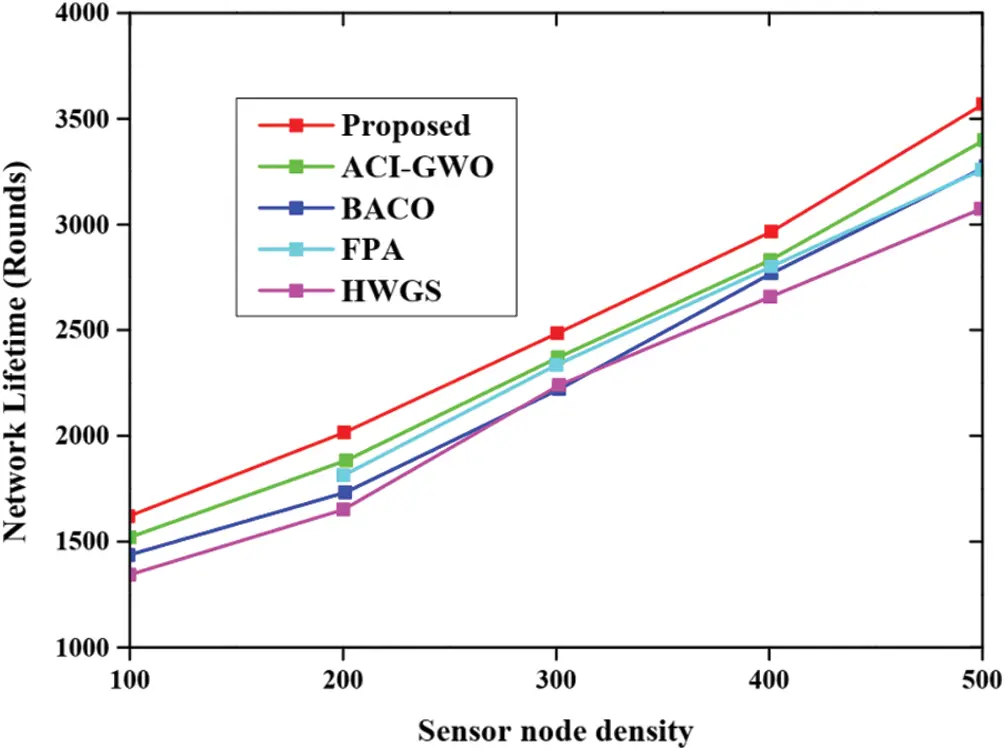Figure 3: Analysis of network lifetime

The comparative graphical analysis of alive node present in the network concerning the diverse routing approaches namely ACI-GWO, BACO, FPA, HGWS as well as the proposed approach is presented in Fig. 4. The figure represented below provides the analysis based on the total number of alive nodes during the processing of the set of rounds. The experimental evaluation is conducted and the analysis demonstrated that our proposed approach has completed 3500 rounds under alive circumstances. But the other existing approaches while completing 3500 rounds the total number of alive nodes is decreased. This indicates that the proposed approach provides better performances with a high number of alive nodes.Figure 4: Analysis based on total number of alive nodes

Fig. 5 presents the comparative analysis based on dead nodes in the network during the processing of set of rounds. In general, the sensor node exhibit in the network dies during task processing. From the graphical analysis it is noted that after the processing of almost 2000 nodes, the existing approaches lost only less number of dead nodes. But the proposed approach lost more dead nodes while completing 3500 rounds. This demonstrates that the proposed approach achieved high performance than other approaches.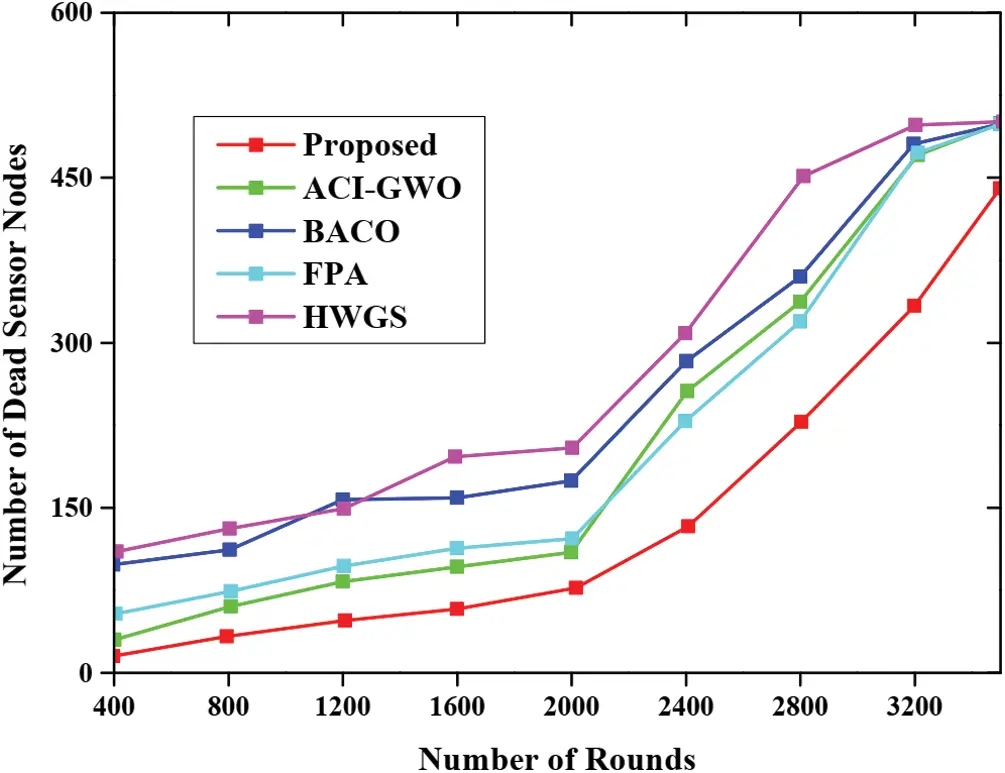Fig. 6 depicts the comparison performance based on energy consumption of the proposed approach with respect to various other existing approaches namely ACI-GWO, BACO, FPA, HGWS. Here, the energy is employed for the processing of tasks containing a diverse number of nodes. From the graphical analysis, the energy consumption of the proposed approach is very low; whereas, in other existing approaches, the energy consumed is very high. This implies that the performances are higher than other approaches. The comparative analysis for the throughput of various approaches along with the proposed approach is represented in Fig. 7. Here, the graphical analysis is plotted for the throughput value and the sensor node density. The experimental analyses are conducted for each respective approach and the comparative analysis is done. Therefore, from the evaluation results, it is clear that the data is transferred at a very high speed in the case of proposed approaches. But the data transfer seems bit lower in other existing approaches.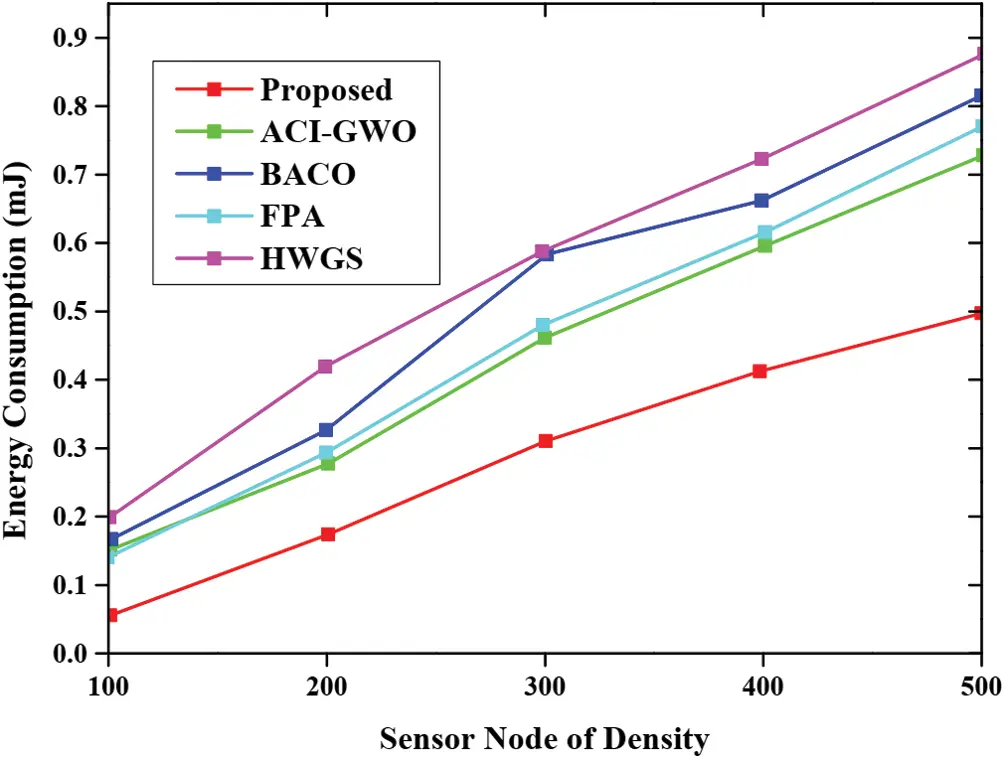Figure 6: Energy consumption analysisFigure 7: Throughput analysis

Fig. 8 presents the pictorial representation for the packet delivery ratio for the proposed approach with respect to various other existing approaches namely ACI-GWO, BACO, FPA and HGWS. From the graphical analysis, the packet is delivered successfully for the proposed approach whereas the packet delivery is low for other existing approaches. Similarly, Fig. 9 presents the graphical analysis based on routing overhead and the analysis revealed that the proposed approach provides minimum routing overhead than other approaches.Figure 8: Evaluation for packet delivery ratio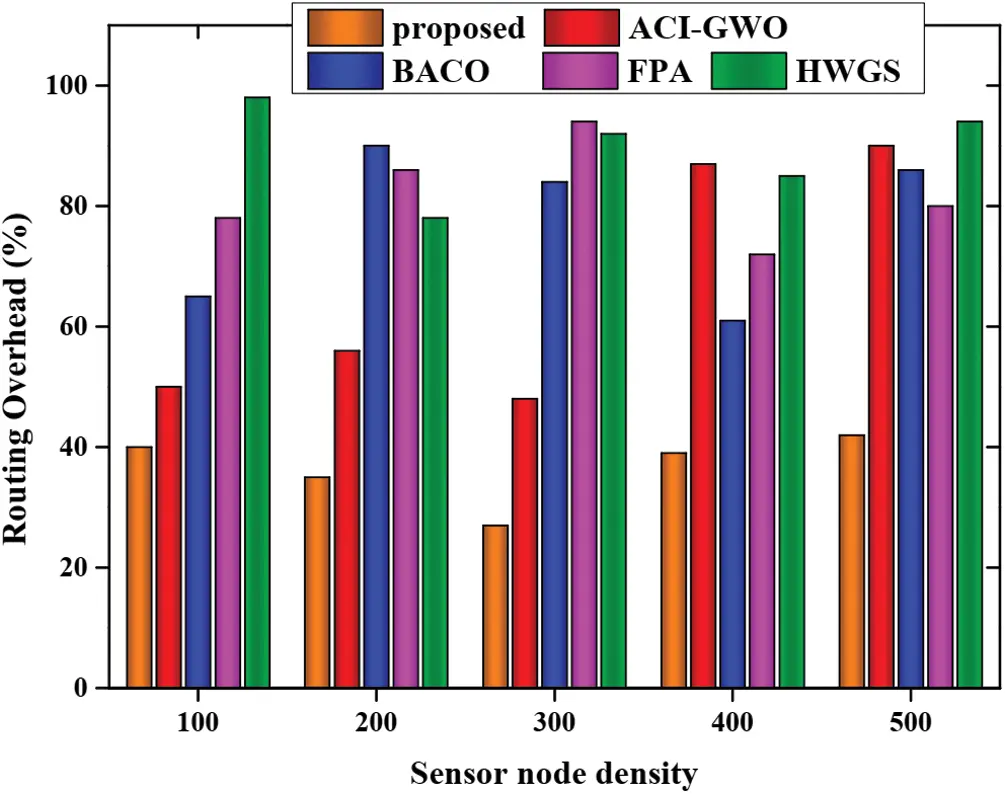7  Conclusion

In WSN, the selection of cluster head and generation of the optimal route is considered as a challenging task. This study considers both distance and energy to develop energy-efficient WSN since the energy consumption of every node depends significantly on the distance among the nodes. Thus the energy consumption is in direct proportion with the distance among the sensor node. The cluster-based routing protocol provides an effective way to minimize the energy consumption and the total number of messages transmitted to the base station or the sink node. In the proposed approach, the CH is selected effectively using M-GEO algorithm. The selection of CH is based on employing node degree, residual energy, the distance among the nearby sensors, centrality of sensor nodes as well as distance among CH and sink nodes. Additionally, a yellow saddle goatfish (YSG) optimization algorithm is employed in generating the optimal routing path from the cluster head to the base station that detects the short routing path to minimize energy consumption. Finally, the proposed energy-efficient routing protocol approach is investigated by employing various parameters and the experimental analyses are conducted and the comparative analysis is made concerning the diverse routing approaches namely ACI-GWO, BACO, FPA, HGWS as well as the proposed approach. Thus the obtained outcome revealed that the proposed approach achieved high performance than other routing-based approaches. The conclusion of the proposed approach also directs the future study to enhance the robustness of the system.

Funding Statement: The authors received no specific funding for this study.

Conflicts of Interest: The authors declare that they have no conflicts of interest to report regarding the present study.

## References

1. N. Moussa and A. E. B. El Alaoui, “An energy-efficient cluster-based routing protocol using unequal clustering and improved ACO techniques for WSNs,” Peer-to-Peer Networking and Applications, vol. 14, no. 3, pp. 1334–1347, 202
2. S. Sankar, S. Ramasubbareddy, F. Chen and A. H. Gandomi, “Energy-efficient cluster-based routing protocol in internet of things using swarm intelligence,” in 2020 IEEE Symp. Series on Computational Intelligence (SSCI), IEEE, University of New South Wales, Canberra Campus, pp. 219–224, 2020.
3. N. M. Shagari, M. Y. Idris, R. B. Salleh, I. Ahmedy, G. Murtaza et al., “Heterogeneous energy and traffic aware sleep-awake cluster-based routing protocol for wireless sensor network,” IEEE Access, vol. 8, pp. 12232–52, 2020.
4. S. Al-Khammasi, D. Alhelal and N. S. Ali, “Energy efficient cluster-based routing protocol for dynamic and static nodes in wireless sensor network,” Telkomnika, vol. 16, no. 5, pp. 1974–1981, 2018.
5. P. S. Rathore, J. M. Chatterjee, A. Kumar and R. Sujatha, “Energy-efficient cluster head selection through relay approach for WSN,” The Journal of Supercomputing, vol. 77, pp. 7649–7675, 2021.
6. Q. Ren and G. Yao, “An energy-efficient cluster head selection scheme for energy-harvesting wireless sensor networks,” Sensors, vol. 20, no. 1, pp. 187, 2020.
7. C. Jothikumar, R. Venkataraman, T. Sai Raj and R. Selva, “An energy efficient cluster-based routing approach to minimize energy consumption using CORP in wireless sensor networks,” Journal of Intelligent & Fuzzy Systems, vol. 36, no. 6, pp. 5835–5844, 2019.
8. V. Sundararaj, “An efficient threshold prediction scheme for wavelet based ECG signal noise reduction using variable step size firefly algorithm,” The International Journal of Intelligent Systems, vol. 9, no. 3, pp. 117–126, 2016.
9. M. M. Gowthul Alam and S. Baulkani, “Geometric structure information based multi-objective function to increase fuzzy clustering performance with artificial and real-life data,” Soft Computing, vol. 23, no. 4, pp. 1079–1098, 201
10. V. Sundararaj, “Optimised denoising scheme via opposition-based self-adaptive learning PSO algorithm for wavelet-based ECG signal noise reduction,” International Journal of Biomedical Engineering and Technology, vol. 31, no. 4, pp. 325, 2019.
11. M. M. Gowthul Alam and S. Baulkani, “Local and global characteristics-based kernel hybridization to increase optimal support vector machine performance for stock market prediction,” Knowledge and Information Systems, vol. 60, no. 2, pp. 971–1000, 2019.
12. V. Sundararaj, S. Muthukumar and R. S. Kumar, “An optimal cluster formation based energy efficient dynamic scheduling hybrid MAC protocol for heavy traffic load in wireless sensor networks,” Computers & Security, vol. 77, pp. 277–288, 2018.
13. S. Vinu, “Optimal task assignment in mobile cloud computing by queue based ant-bee algorithm,” Wireless Personal Communications, vol. 104, no. 1, pp. 173–197, 2019.
14. M. R. Rejeesh, “Interest point based face recognition using adaptive neuro fuzzy inference system,” Multimedia Tools and Appications, vol. 78, no. 16, pp. 22691–22710, 2019.
15. M. R. Rejeesh and P. Thejaswini, “MOTF: Multi-objective optimal trilateral filtering based partial moving frame algorithm for image denoising,” Multimedia Tools and Appications, vol. 79, no. 37, pp. 28411–28430, 2020.
16. M. Azath, R. W. Banu and A. N. Madheswari, “Improving fairness in network traffic by controlling congestion and unresponsive flows,” in Int. Conf. on Network Security and Applications, Springer, Berlin, Heidelberg, pp. 356–363, 2011.
17.  M. Azath, A. N. Madheswari and R. W. Banu, “Core-stateless fair queuing for fair bandwidth allocations in internet,” in 2009 Int. Conf. on Advances in Computing, Control, and Telecommunication Technologies, Bangalore, India, IEEE, pp. 596–598, 2009.
18. S. Nisha and A. N. Madheswari, “Prevention of phishing attacks in voting system using visual cryptography,” in 2016 Int. Conf. on Emerging Trends in Engineering, Technology and Science (ICETETS), Pudukkottai, India, IEEE, pp. 1–4, 2016.
19. B. M. Sahoo, H. M. Pandey and T. Amgoth, “GAPSO-H: A hybrid approach towards optimizing the cluster-based routing in wireless sensor network,” Swarm and Evolutionary Computation, vol. 60, pp. 100772, 2021.
20. B. M. Sahoo, T. Amgoth and H. M. Pandey, “Particle swarm optimization-based energy efficient clustering and sink mobility in heterogeneous wireless sensor network,” Ad Hoc Networks, vol. 106, pp. 102237, 20
21. K. M. Awan, A. Ali, F. Aadil and H. N. Qureshi, “Energy efficient cluster-based routing algorithm for wireless sensors networks,” in 2018 Int. Conf. on Advancements in Computational Sciences (ICACS), Lahore, Pakistan, IEEE, pp. 1–7, 2018.
22. P. Maheshwari, A. K. Sharma and K. Verma, “Energy efficient cluster-based routing protocol for WSN using butterfly optimization algorithm and ant colony optimization,” Ad Hoc Networks, vol. 110, pp. 102317, 2021.
23. R. Sharma, M. Nitin and B. S. Sohi, “Flower pollination algorithm-based energy-efficient stable clustering approach for WSNs,” International Journal of Communication Systems, vol. 33, no. 7, pp. e4337, 2020.
24. K. N. Qureshi, M. U. Bashir, J. Lloret and A. Leon, “Optimized cluster-based dynamic energy-aware routing protocol for wireless sensor networks in agriculture precision,” Journal of Sensors, vol. 2020, Article ID 9040395, 2020. https://doi.org/10.1155/2020/9040395.
25. T. Vaiyapuri, V. S. Parvathy, V. Manikandan, N. Krishnaraj, D. Gupta et al., “A novel hybrid optimization for cluster-based routing protocol in information-centric wireless sensor networks for IoT based mobile edge computing,” Wireless Personal Communications, pp. 1–24, 2021. https://doi.org/10.1007/s11277-021-08088-w.
26. V. Chauhan and S. Soni, “Mobile sink-based energy efficient cluster head selection strategy for wireless sensor networks,” Journal of Ambient Intelligence and Humanized Computing, vol. 11, no. 11, pp. 4453–4466, 2020.
27. L. Nagarajan and S. Thangavelu, “Hybrid grey wolf sunflower optimisation algorithm for energy-efficient cluster head selection in wireless sensor networks for lifetime enhancement,” IET Communications, vol. 15, no. 3, pp. 384–396, 2021.
28. T. Ahmad, M. Haque and A. M. Khan, “An energy-efficient cluster head selection using artificial bee's colony optimization for wireless sensor networks,” in Advances in Nature-Inspired Computing and Applications, Cham: Springer, pp. 189–203, 2019.
29. S. Balaji, E. G. Julie and Y. H. Robinson, “Development of fuzzy based energy efficient cluster routing protocol to increase the lifetime of wireless sensor networks,” Mobile Networks and Applications, vol. 24, no. 2, pp. 394–406, 2019.
30. J. G. Lee, S. Chim and H. H. Park, “Energy-efficient cluster-head selection for wireless sensor networks using sampling-based spider monkey optimization,” Sensors, vol. 19, no. 23, pp. 5281, 2019.
31. R. Sharma, V. Vashisht and U. Singh, “eeTMFO/GA: A secure and energy efficient cluster head selection in wireless sensor networks,” Telecommunication Systems, vol. 74, pp. 253–268, 2020.
32. D. L. Reddy, C. Puttamadappa and H. N. Suresh, “Merged glowworm swarm with ant colony optimization for energy efficient clustering and routing in wireless sensor network,” Pervasive and Mobile Computing, vol. 71, pp. 101338, 2021.
33. D. Mehta and S. Saxena, “MCH-EOR: Multi-objective cluster head-based energy-aware optimized routing algorithm in wireless sensor networks,” Sustainable Computing: Informatics and Systems, vol. 28, pp. 100406, 2020.
34. L. Ye, Y. Hong, M. Liao, B. Wang and H. Peng, “Recent advances in flexible fiber-shaped metal-air batteries,” Energy Storage Mater, vol. 28, pp. 364–374, 2020.
35. J. Li and H. Zhang, “Thermal characteristics of power battery module with composite phase change material and external liquid cooling,” Int. J. Heat Mass Transfer, vol. 156, pp. 119820, 2020.
36. A. Mohammadi-Balani, M. D. Nayeri, A. Azar and M. Taghizadeh-Yazdi, “Golden eagle optimizer: A nature-inspired metaheuristic algorithm,” Computers & Industrial Engineering, vol. 152, pp. 107050, 2021.
37. M. S. Braik, “Chameleon swarm algorithm: A bio-inspired optimizer for solving engineering design problems,” Expert Systems with Applications, vol. 174, pp. 114685, 2021.
38. D. Zaldivar, B. Morales, A. Rodríguez, A. Valdivia-G, E. Cuevas et al., “A novel bio-inspired optimization model based on yellow saddle goatfish behavior,” Biosystems, vol. 174, pp. 1–21, 2018.This work is licensed under a Creative Commons Attribution 4.0 International License, which permits unrestricted use, distribution, and reproduction in any medium, provided the original work is properly cited.